### Lab Report on Acid-Base Titrations of ammonia, acetic acid, NaOH and HCl

The lab report below was submitted as part of the coursework for CM1101 Basic Physical Chemistry. Please do not plagiarise from it as plagiarism might land you into trouble with your university. Do note that my report is well-circulated online and many of my juniors have received soft copies of it. Hence, please exercise prudence while referring to it and, if necessary, cite this webpage.

1. Aim: To determine the weight-to-volume percent of acetic acid present in commercial vinegar and the weight-to-volume percent of ammonia present in window cleaners. Acid-base titrations can be conducted using the above samples against standardized aqueous NaOH and standardized aqueous HCl solutions respectively.
2. Introduction:
Acetic acid, commonly known as ethanoic acid CH3COOH, is found in commercial vinegar. It is a weak organic acid that gives vinegar its characteristic pungent smell, sour taste and slight acidity. Being an acid, vinegar is used as a mild disinfectant in cleaning agents as well as a food preservative. Ammonia, NH3, is used in household and industrial cleaning agents due to its ability to solubilize grease.  In this experiment, we shall use acid-base titration to determine the weight-to-volume percentage of acetic acid and ammonia. An acid-base titration is a process of obtaining quantitative information of a sample using an acid-base reaction by reacting with a certain volume of reactant whose concentration is known. A suitable indicator for determining the equivalence point is used to indicate the end point of an acid-base titration. To achieve accurate results, multiple titrations will be carried out until duplicate determinations agree to within 0.05mL of each other.
4. Results & Calculations:
4.1 Standardization of NaOH
Molarity of KHC8H4O4 is 0.05044 moldm-3   (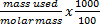=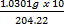= 0.05044 moldm-3)
 Titration 1 2 3 Final volume of titrant/cm3 5.00 10.00 14.80 Initial volume of titrant/cm3 0.00 5.00 10.00 Volume of titrant used/cm3 5.00 5.00 4.80
KHC8H4O4 (aq)   +  NaOH (aq)                      KHC8H4O3 - Na+  (aq)  +  H2O (l)
Average volume of NaOH titrate used = ½ (5.00 + 5.00)
= 5.00 cm3
No. of moles of KHC8H4O4   reacted = 0.01 × 0.05044
= 5.044 x 10-4 mol

From the equation, mole ratio of NaOH ≡ KHC8H4O4   is 1:1,
No. of moles of NaOH reacted                 = 5.044 x 10-4 mol
Concentration of NaOH = moles of NaOH/ volume of NaOH
= 5.044 x 10-4 /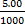= 0.1009 moldm-3
Molarity of NaOH is: 0.1009moldm-3
4.2 Standardization of HCl
 Titration 1 2 3 Final volume of titrant/cm3 10.80 10.75 10.70 Initial volume of titrant/cm3 0.00 0.00 0.00 Volume of titrant used/cm3 10.80 10.75 10.70
HCl (aq)   +  NaOH (aq)                     NaCl (aq)  +  H2O (l)
Average NaOH titrate used = ½ (10.70 + 10.75)
= 10.73 cm3
No. of moles of NaOH   reacted = (10.73 ÷ 1000) × 0.1009
= 1.082 x 10-3 mol

From equation, mole ratio of NaOH ≡ HCl   is 1:1,
no. of moles of HCl reacted                     = 1.082 x 10-3 mol

Hence concentration of HCl = 1.082 x 10-3 (1000 ÷ 10.00)
= 0.1082 moldm-3

Molarity of HCl is: 0.1082 moldm-3

4.3 Determination of acetic acid in vinegar
 Titration 1 2 3 Final volume of titrant/cm3 12.15 12.20 12.20 Initial volume of titrant/cm3 0.00 0.00 0.00 Volume of titrant used/cm3 12.15 12.20 12.20
CH3COOH (aq)   +  NaOH (aq)                     CH3COO-Na+ (aq)  +  H2O (l)

Average volume of NaOH titrate used = ½ (12.20 + 12.20)
= 12.20 cm3
No. of moles of NaOH reacted             = (12.20  ÷ 1000) × 0.1009
= 1.231 x 10-3 mol

From equation, mole ratio of NaOH ≡ CH3COOH   is 1:1,
no. of moles of CH3COOH reacted           = 1.231 x 10-3 mol

No. of moles of CH3COOH in volumetric flask (diluted) = (100 ÷ 10) × 1.231 x 10-3
= 1.231 x 10-2 mol
No. of mole of CH3COOH in 25mL (undiluted) = 1.231 x 10-2 mol
Molarity of acetic acid = (1.231 x 10-2 mol) ÷(25 x 10-3)=0.4924 moldm-3
Molar mass of CH3COOH = 2(12.01) + 4(1.0079) + 2(16.00)
= 60.0516 g mol-1

Therefore, weight-volume percentage of CH3COOH in vinegar
= (0.4924 x 60.0516 ÷ 1000) × 100% = 2.96%

Percentage of acetic acid in the vinegar is: 2.96%
Indicator used: phenopthalein

4.4 Determination of ammonia in window cleaner
 Titration 1 2 3 Final volume of titrant/cm3 4.85 9.65 4.85 Initial volume of titrant/cm3 0.00 4.85 0.00 Volume of titrant used/cm3 4.85 4.80 4.85
NH3 (aq)   +  HCl (aq)                     NH4+Cl- (aq)

Average volume of HCl titrate used = ½ (4.85 + 4.85)
= 4.85 cm3
No. of moles of HCl reacted = 0.1082 x (4.85 x10-3)
= 5.248 x 10-4 mol

From equation, mole ratio of HCl ≡ NH3   is 1:1,
no. of moles of NH3 reacted = 5.248 x 10-4 mol
No. of moles of NH3 in volumetric flask (diluted) = (100 ÷ 10) × 5.248 x 10-4 mol
= 5.248 x 10-3 mol
No. of mole of NH3 in 10mL (undiluted) = 5.248 x 10-3 mol
Concentration of NH3   = (5.248 x 10-3) ÷ (10 x 10-3) = 5.248 x 10-1 moldm-3

Molar mass of NH3 = 14.01 + 3 (1.0079)
= 17.0337 g mol-1

Therefore, weight-volume percentage of NH3 in window cleaner
= (17.0337 x 5.248 x 10-1)/ 103 × 100%
= 0.894%

Percentage of ammonia in the window cleaner is: 0.894%
Indicator used: methyl red

5. Discussion:
The main reaction in the series of acid-base titrations is neutralization. During neutralization, the acid and base reacts to form a salt and water (i.e. H+ + OH-          H2O). As the all the reactants are colourless, an appropriate indicator must be added to indicate the colour change at the end point.

 For Experiment 3.3, the indicator phenolphthalein was chosen as it was a weak acid-strong base titration, as acetic acid is a weak acid and NaOH is a strong base. At equivalence point, the resulting solution contains only CH3COONa. Thus salt hydrolysis occurs and the pH at equivalence point is > 7. Phenolphthalein changes colour at a pH range of 8.2 – 10.0. The (pH)end point falls within the pH for colour change of phenolphthalein in the titration. The colour of phenolphthalein changes explicitly from pink to colourless and the colour change is over a narrow pH range. This further enhances its suitability as an indicator for this titration. Salt hydrolysis that raises equivalence point to > 7 pH : CH3COO-  +  H2O          CH3COOH  +  OH-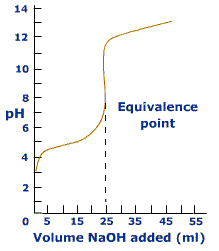* The pH titration curve of weak acid (CH3COOH) and strong base (NaOH)

 However, for Experiment 3.4 a different indicator was chosen. Methyl red was used instead as it was a case of a strong acid-weak base titration, as HCl is a strong acid and NH3 is the weak base. At equivalence point, the resulting solution contains only NH4Cl. Thus salt hydrolysis occurs and the pH at equivalence point is < 7. Methyl red changes colour over a pH range of 4.8 – 6.0. The (pH)end point falls within the pH range for colour change of methyl red in this titration. The colour of methyl red changes explicitly from red to yellow and the colour change is over a narrow pH range. This further enhances its suitability as an indicator for this titration. Salt hydrolysis that lowers equivalence point to < 7 pH : NH4Cl  +  H2O           NH3  +  H3O+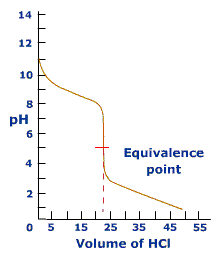* The pH titration curve of weak base (NH3) and strong acid (HCl)
Strong acid-weak base and weak acid-strong base titrations are employed in this experiment instead of weak acid-weak base titrations as the hydrolysis of a salt of a weak acid-weak base is incomplete. The hydrolysis of the conjugate base results in an acidic solution and at the same time, hydrolysis of the conjugate acid of the salt gives rise to a basic solution. Hence, the resulting solution at equivalence point can be neutral, acidic or basic depending on which hydrolysis occurs at a greater extent. This means that calculations have to be done preceding the titration in order to select the appropriate indicators. Therefore, weak acid-weak base titration is not commonly used to determine the quantitative information of an unknown sample.
While titrating, the conical flask has to be swirled constantly throughout to ensure that the contents are mixed evenly. Also, some titrant may drip onto the sides of the conical flask and may not react with the solution in the conical flask. This reduces the accuracy of the results as extra titrant would be used to achieve the equivalence point. To prevent this, deionised water can be used to wash down the unreacted titrant when nearing the equivalence point and the conical flask should be swirled before continuing with the titration. To achieve consistent results, one important factor is to add in the titrant in a drop wise manner before the equivalence point.

Deionised water was used not only for diluting the sample but also for washing the apparatus, instead of using tap water. This is because deionized water is pH neutral and will not negatively affect the results of the acid-base titrations. This is important as acid-base titrations are pH sensitive reactions.

Multiple titrations were carried out until duplicate determinations agree to within 0.05mL of each other. This ensures that the results are not once-off outliers. By averaging the two most consistent and accurate results, the probability of incurring random errors was accounted for and decreased.

Other factors that would affect the accuracy of the results include parallax error when taking the readings and making sure that no air bubbles are present within the pipette and burette while titrating.

6. Conclusion:
Using acid-base chemistry, repeated titrations of vinegar sample and window cleaner sample was carried out against standardized solutions of NaOH and HCl respectively. Through stoichiometric relations, the concentration and weight-to-volume percentage of acetic acid in vinegar and ammonia in window cleaner can be found. The percentage of acetic acid in the vinegar sample studied was deduced to be 3.96%. The percentage of ammonia in the window cleaner sample is 0.894%.

7. References:
8. Exercises
1. Given that 1mL of NH3 (aq) contains 0.899g of NH3,:
Mass of solute of NH3 (g) in 1mL = (28 ÷ 100) × 0.899 = 0.25172 g
No. of moles of NH3 in 0.2517g or 1mL = 0.2517 ÷ (14.00 + 3.00) = 0.01481 mol
Hence, the concentration of NH3 = 0.01481 × (1000 ÷ 1)     = 14.8M (3s.f.)

2i. Given that 1mL of HCl (l) contains 1.18g of HCl:
Mass of solute of HCl (g) in 1mL = (37 ÷ 100) × 1.18 = 0.4366 g
No. of moles of HCL in 0.4366g or 1mL = 0.4366 ÷ (35.50 + 1.00)  = 0.01196 mol
Hence, concentration of HCl = 0.01196 × (1000 ÷ 1)      = 12.0M (3s.f.)

2ii.
Volume of HCl in 0.3150 mol = (0.3150 ÷ 11.96) × 100 =26.29 cm3
Mass of HCl in 0.3150 mol = 26.29 x 1.18                   = 31.03g

3.   SO2 (g)  +  H2O2 (aq)          H2SO4 (aq)  Equation 1H2SO4 (aq)  +  2NaOH (aq)          Na2SO4 (aq)  +  H2O (l)  Equation 2From equation 1: no. of moles of NaOH reacted = (46.75 ÷ 1000) × 0.1251
= 0.005848 mol
Since H2SO4 ≡ 2NaOH, no. of moles of H2SO4 reacted = ½ (0.005848)
= 0.002924 mol
From equation 2, H2SO4 ≡ SO2, no. of moles of SO2 = 0.002924 mol
Since C6H8N2O2S ≡ SO2, experimental mass of C6H8N2O2S reacted
= 0.5034 g
Hence, percentage purity of C6H8N2O2S = (0.5034 ÷ 0.5136) × 100%
= 98.04%
4. HCl (aq)  +  NaOH (aq)          NaCl (aq)  +  H2O (l)No. of moles of NaOH reacted = (37.85 ÷ 1000) × 0.05992
= 0.002268 mol
Since HClexcess ≡ NaOH, no. of moles of HClreacted = [(50 ÷ 1000) × 0.09552] – 0.002268
= 0.004776 – 0.002268
= 0.002508 mol
HCl (aq)  +  NH3 (g)          NH4Cl (aq)
Since HCl ≡ NH3, no. of moles of NH3 = 0.002508 mol
Hence mass of N present in sample = Ar of N × no. of moles of NH3 = 0.002508(14.01)
= 0.03514 g
Mass of protein derived from mass of N = (100 ÷ 17.54)% × 0.03514
= 0.2003 g
Therefore, % w/w protein in cereal sample = (0.2003 ÷ 1.2846) × 100%
= 15.59%

1.1.Sometime in 2011, I would say :]

2.btec diploma studentOctober 12, 2015 at 9:22 PM

who owns the content?

1.3.Is there any ethical issue of conducting an experiment like this?

1.Hi, this is a typical acid-base reaction. Just observe safety rules at all time!

Ethics wise, I think there should be no problem :]

4.ummm hey is it ok if i used your document as a reference material for my research???

1.Definitely!

5.It's probably interesting but only for physicists and chemists. If I would have to write a coursework like this one, I would buy cheap coursework

6.Listen can I copy paste this for my school lab report

1.